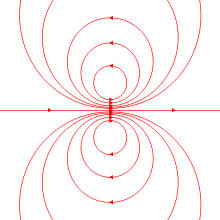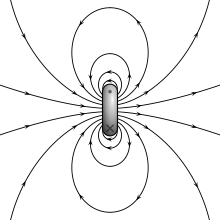Magnetic Moment for IIT JEE

Magnetic Dipole Moment JEE Physics - Definition and Equation

Magnetic Moment is all about the magnet so we can define this as a determination of magnet’s tendency which gets arranged by a magnetic field. If we want to elaborate this definition in general terms, we can say that Magnet Moment of a magnet is that quantity which finds the force that a magnet is capable of applying on electric currents and also the torque that the magnetic field will exert on it. There are numerous examples of the Magnetic Moment which we encounter like, an electron, a planet or a molecule and a bar magnet and so on. Today through this article we try to find out magnetic moment formula.

In very simple terms Magnetic Moment is explained as a vector that has direction as well as magnitude. Magnetic Moment has the direction that starts from the South Pole to the North Pole of a magnet. As you all know that every magnet has 2 poles which are North Pole and South Pole. Here we can explain the meaning of Magnet length. Magnet length is the distance between the north and south poles of a magnet or a magnetic dipole which is given as the 2 µ.

Suppose m is the power of any kind of magnetic pole so the vector M provides the magnet’s magnetic dipole moment. You can understand this with below given articulation:

M = m / 2 µ

Here, m is the strength of any magnetic dipole

Moreover, µ is magnet length

Here we must notice that the magnet which creates the magnetic field is directly proportional to its magnetic moment. The term magnetic moment is all about the magnetic dipole moment of the system. The dipole part and parcel of one object’s magnetic field is regularity about the direction of its magnetic dipole moment and it reduces as the inverse cube of the distance from the object. You can call Magnetic moment as a magnetic dipole moment (because it’s another name of magnetic moment) the way an object reacts to its magnetic field decides magnetic moment’s positive direction. Objects tend to place themselves in such a way that the magnetic moment vector becomes parallel to the magnetic field lines.

Magnetic Moment’s Possible Alternative Definitions-

Suppose there is a choice as to whether by magnetic field we choose H or B if someone chooses to define the magnetic moment as the maximum torque experienced in the unit external to the magnetic field. So we have two equations to define the magnetic moment, which is given below:

τ = p1H
τ = p2B

The generation of Magnetic Moment basically includes two ways:

• 1. The Motion of Electric Charge

• 2. Spin Angular Momentum

• Let’s take one example. If there is a loop of wire in which the current is running through, it will have a magnetic moment which is proportional to the current. Moreover the area of the loop points in the direction of anyone’s right thumb when the fingers are curling in the direction of the current.

The SI definition of Magnetic Moment-

The magnet will experience a torque if it is placed in an external magnetic field B. The Torque’s magnitude always depends on the orientation of the magnet with respect to the magnetic field. The magnet experiences the greatest torque in two oppositely-directed orientations; therefore, magnitude of the magnetic moment is defined as “the magnet when placed in a unit external magnetic field experiences the maximum torque. The below-mentioned equation displays the magnitude and direction of the torque-

τ = p×B
N m T-1 is the SI unit for the magnetic moment

The torque experienced in a magnetic field, when an electric current I flows in a plane coil area A (it is boldface because that area is a vector quantity), is given by

τ = IA×B

So we can denote the magnetic moment of the coil as:

p = IA

Therefore, a correct SI unit for the magnetic moment could also be A m2 , however, expecting that the concept of “current in a coil” requires to be a highlight in a particular context, so it is better to be stick to N m T-1.

Although the unit J T-1 is also dimensionally correct still it is good to limit the unit “joule” to energy or work, instead of the use of N m for torque. However, these are conceptually different and dimensionally similar. Expressing magnetic moments in Me V T-1 is the occasional practice which is seen in atomic physics, though it is not completely appropriate. It may sometimes seem to be in a field in which moment and masses are often conveniently expressed in Me V/c2 and Me V/c. A unit T m3, which is seen for the magnetic moment often, is not dimensionally correct for magnetic moment, that’s why whichever quantity has been expressed by the T m3 is not the standardized defined concept of the magnetic moment.

The equation which defines the magnetization M of material is:

B = µ0(H + M)

The Magnet’s Magnetic Field on the Equator-

A plane (means the “equator” of a magnet), normal to its magnetic moment vector, is passing through the mid-point of the magnet. A series of terms of successively higher powers of 1/r expresses the magnetic field at a point at a distance r on the magnet’s equator moreover the higher powers become negligible and that’s why the magnitude of the magnetic field produced by the magnet at a large distance from a small magnet is given by:

B = µ0 / 4 π p / r3

Magnetic Dipole-

We generally define magnetic dipole as a dipole which defines the limit of a pair of poles or a current loop because the dimension of sources is diminished to zero albeit the moment is kept as constant. These limits are the same when these limits apply to the fields far away from the sources. Although for the internal field the two models are different. The topic of Magnetic Dipole Moment is the most influential topic for the aspirants who are about to embark on the preparation of IIT JEE since it initiates many other topics.

We can understand this topic in some general term. In general term, Magnetic Dipole is a kind of minute magnet of microscopic to subatomic dimensions which is equivalent to a flow of electric charge around a loop. Some of few practical examples of magnetic dipoles are electrons spinning on their axes, electrons circulating around atomic nuclei and rotating atomic nuclei which are positively charged. Sometimes a given type of atom might not be a magnetic dipole if some of these effects get cancelled. Although in other cases if they don’t fully cancel as are iron atoms, the atom is a permanent magnetic dipole. For the formation of a ferromagnetic domain many millions of iron atoms spontaneously lock into the same alignment moreover they also constitute a magnetic dipole. Few examples of macroscopic magnetic dipoles are magnetic compass needles and bar magnets.

The magnitude of the dipole moment is proportional to the current multiplied by the size of the enclosed area in the case when we consider a magnetic dipole as a current loop. A vector is a mathematical representation of the direction of the dipole moment. It is perpendicularly away from the side of the surface which is enclosed by the counter-clockwise path of positive charge flow.

Magnetic Dipole Moment-

The area or energy of the current time is divided by magnetic flux density. These dimensions have the magnetic dipole moments. When it is about the SI systems and centimetre-gram-second-ampere the exact unit for dipole moment is ampere-square meter. If it’s about the unit is the erg (unit of energy) per gauss (unit of magnetic flux density) so the centimetre-gram-second electromagnetic system. One ampere-square meter is equal to around one thousand ergs per gauss. The Bohr magneton which is equal to 9.27 * 10-24 ampere-square meter, is absolutely a fitting unit of the electron’s magnetic dipole moment. The nuclear magneton which is equal to 5.051 * 10-27 ampere-square meter is a similar unit for magnetic moments of neutrons, protons and nuclei.

Magnetic Dipole Moment Produces External Magnetic Field-A system produces a dipolar magnetic field. It means that it includes a net magnetic dipole moment m. The net magnetic field that may show comparatively high-order multipole components means that it is created by the system. However, it is intriguing to notice that in the end the system’s dipolar component dominates the magnetic field at distances far away from it and they fall out quite rapidly with distance.

Dipole’s Internal Magnetic Field-The most important thing which needs to be acknowledged is that two models for a dipole provide the exact same result for the magnetic field far from the source. The magnetic field is in the opposite direction to the magnetic moment between the two poles.